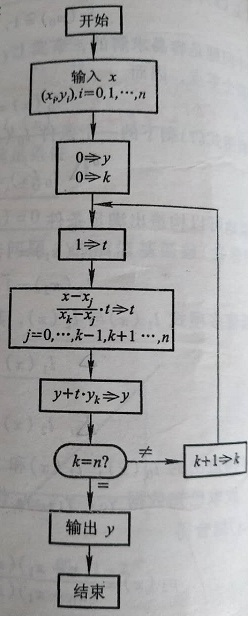• 拉格朗日插值公式 背公式吧，没什么好说的了。。。 假装$$P$$是一个关于$$x$$的$$n$$次多项式，我们已经知道了$$P(i),i\in[0,n]$$的值。 $P(x)=\sum_{i=0}^n(-1)^{n-i}P(i)\frac{x(x-1)(x-2)...(x-n)}{(n-i)!i!(x-i... 拉格朗日插值公式 背公式吧，没什么好说的了。。。 假装$$P$$是一个关于$$x$$的$$n$$次多项式，我们已经知道了$$P(i),i\in[0,n]$$的值。\[P(x)=\sum_{i=0}^n(-1)^{n-i}P(i)\frac{x(x-1)(x-2)...(x-n)}{(n-i)!i!(x-i)}$
上面这个东西是拉格朗日插值公式的特殊情况。
一般情况下是任意的$$n+1$$个给定的点$$x_i$$以及值$$P(x_i)$$
丢下公式就跑$P(x)=\sum_{i=0}^{n}P(x_i)\prod_{j=0,j\ne i}^{n}\frac{x-x_j}{x_i-x_j}$
模板戳这里

转载于:https://www.cnblogs.com/cjyyb/p/9392388.html
展开全文• 推导抛物线插值的拉格朗日插值公式 发现历程 ​ 在数值分析中，拉格朗日插值法是以法国十八世纪数学家约瑟夫·路易斯·拉格朗日命名的一种多项式插值方法。许多实际问题中都用函数来表示某种内在联系或规律，而不少...
推导抛物线插值的拉格朗日插值公式
发现历程
​	 在数值分析中，拉格朗日插值法是以法国十八世纪数学家约瑟夫·路易斯·拉格朗日命名的一种多项式插值方法。许多实际问题中都用函数来表示某种内在联系或规律，而不少函数都只能通过实验和观测来了解。如对实践中的某个物理量进行观测，在若干个不同的地方得到相应的观测值，拉格朗日插值法可以找到一个多项式，其恰好在各个观测的点取到观测到的值。这样的多项式称为拉格朗日（插值）多项式。数学上来说，拉格朗日插值法可以给出一个恰好穿过二维平面上若干个已知点的多项式函数。拉格朗日插值法最早被英国数学家爱德华·华林于1779年发现，不久后（1783年）由莱昂哈德·欧拉再次发现。1795年，拉格朗日在其著作《师范学校数学基础教程》中发表了这个插值方法，从此他的名字就和这个方法联系在一起。
拉格朗日插值法
​	一般地，若已知 在互不相同 n+1 个点 处的函数值（即该函数过 这n+1个点），则可以考虑构造一个过这n+1个点的、次数不超过n的多项式 ,使其满足：
​	要估计任一点ξ，ξ≠xi,i=0,1,2,…,n,则可以用Pn（ξ）的值作为准确值f(ξ)的近似值，此方法叫做“插值法”。
称式（*）为插值条件（准则），含xi(i=0,1,…,n)的最小区间[a,b]，其中a=min{x0,x1,…,xn}，b=max{x0,x1,…,xn}。
​	满足插值条件的、次数不超过n的多项式是存在而且是唯一的。
举个栗子
在平面上有共n个点，现作一条函数f(x) 使其图像经过这n个点。
作法：设集合D(n)是关于点(x,y)的角标的集合，D(n)={0,1,2,…,n-1}作n个多项式Pj(x),j属于D(n)
对于任意k都有使得Pk(n-1)是n-1次多项式，且满足并且Pk（Xk）=1
最后可得形如上式的插值多项式Ln（x）称为拉格朗日（Lagrange）插值多项式。
例如：当n=4时，上面的公式可简化为：这是一个过4个点的唯一的三次多项式。
抛物插值
抛物插值就是通过3个采样点(x0,y0),(x1,y1),(x2,y2)，即只要确定出3个插值基函数即可。
代入三个点到上述的拉格朗日插值定理即可得：
,(x1,y1),(x2,y2)，即只要确定出3个插值基函数即可。
代入三个点到上述的拉格朗日插值定理即可得：展开全文• [数值分析]拉格朗日插值公式 实际问题中碰到的函数f(x)是各种各样的，有的表达式很复杂，有的甚至给不出数学式子，只提供了一些离散数据，譬如某些点上的函数值和导数值。由于问题的复杂性，直接研究函数f(x)可能很...
实际问题中碰到的函数f(x)是各种各样的，有的表达式很复杂，有的甚至给不出数学式子，只提供了一些离散数据，譬如某些点上的函数值和导数值。由于问题的复杂性，直接研究函数f(x)可能很困难。面对这种情况，一个很自然的想法是，设法将所考察的函数f(x)“简单化”，就是说，构造某个简单的函数p(x)作为f(x)的近似函数，然后通过处理p(x)获得关于f(x)的结果。如果要求近似函数p(x)取给定的离散数据，则称之为f(x)的插值函数。
例：已知根号100等于10，根号121等于11，根号144等于12，试用拉格朗日插值公式求解根号115的值。

运行示例：源码：

#include<iostream>
using namespace std;

#define MAX 10

typedef struct Point  //插值节点信息结构体
{
double x;
double y;
}point;

int main(void)
{
int n;   //插值节点个数
double x, item, sum;   //item为每一项插值基函数与其对应函数值的乘积

point p[MAX];   //定义结构体数组

sum = 0;

cout << "请输入插值节点的个数:";
cin >> n;

for (int i = 1; i <= n; i++)   //将节点信息存入结构体数组
{
cout << "请输入第" << i << "个点的坐标:";
cin >> p[i].x;
cin >> p[i].y;
}

cout << "请输入x的值:";   //输入x提示
cin >> x;

for (int i = 1; i <= n; i++)    //外层循环实现对item的累加
{
double denominator = 1;   //分母赋初值为1
double numerator = 1;   //分子赋初值为1

for (int j = 1; j <= n; j++)    //内层循环实现拉格朗日基函数各分子、分母部分的求解
{
if (i == j)
{
continue;
}
denominator *= p[i].x - p[j].x;   //基函数分母
numerator *= x - p[j].x;   //基函数分子
}

item = numerator / denominator * p[i].y;   //求解拉格朗日插值法的每一项
sum += item;   //累加求和
}

cout << "运用拉格朗日基函数插值法求得f(x) ≈ p(x) = " << sum << endl;

return 0;
}



展开全文c++ 数值分析
• 数值计算基础实验之一 拉格朗日插值公式求点的函数值的C语言实现
• 拉格朗日插值公式 流程图如下： python代码实现如下： # coding=gbk; def Data_in(list0,list1,list2): list0=input("请输入离散点的值：").split(" "); for i in range(0,len(list0)): if i%2==0: #...
拉格朗日插值公式
流程图如下：python代码实现如下：
# coding=gbk;
def Data_in(list0,list1,list2):
list0=input("请输入离散点的值：").split(" ");
for i in range(0,len(list0)):
if i%2==0:  #如果是奇数  那么指的也就是x
list1.append(float(list0[i]));
else:  #如果是偶数 那么就是y
list2.append(float(list0[i]));
return list0,list1,list2;

def Lagrange_insert(list1,list2,length,x_in1):
k=0;
y_out=0;
while k<=length-1:
t=1;  j=0;   count=0;  #用来计算多少次
while count!=n-1:  #肯定是只能够N-1次
if j==k:
j+=1;
continue;
t_temp1=x_in1-list1[j];
t_temp2=list1[k]-list1[j];
t=t_temp1/t_temp2*t
count+=1;
y_out+=t*list2[k];
k+=1
return y_out;

temp_list=[]; x=[];  y=[];
temp_list,x,y=Data_in(temp_list,x,y);
n=len(y);   #n用来记录多少组数据

x_in=float(input("请输入想要预测的x值："));
y_predict=Lagrange_insert(x, y, n, x_in);
print(y_predict)


遇事不决，可问春风


展开全文python
• 题目大意：求 ∑i=1nik\...拉格朗日插值法求k次幂之和 这里采用拉格朗日插值法进行求解。 拉格朗日可以通过 k+1k + 1k+1 个点唯一确定一个 kkk 次多项式，它的公式为：f(x)=∑i=1ny[i]∏i≠jx−x[j]x[i]−x...
• # 拉格朗日差值公式 # x, y 按顺序一比一输入 xs = [1.45, 1.36, 1.14] ys = [3.14, 4.15, 5.65] # eval(input()) # 原函数不一定升序 # xs.sort() # ys.sort() x=1.4 Lsum=0 len_xy=len(xs) for i in range...python
• 插值方法 插值方法是用来处理和分析数据的方法，所谓插值就是在所给数据的基础上再插入一些所需的值，但这些值不是随便给出的，而是在已有数据的基础上进行分析，给出的近似值。 插值方法要解决的问题 首先当我们...算法 数据分析
• 线性插值(一次插值）  已知函数f(x)在区间[xk ,xk+1 ]的端点上的函数值yk =f(xk ), yk+1 = f(xk+1 ),求一个一次函数y=P1 (x)使得yk =f(xk ),yk+1 =f(xk+1 ), 其几何意义是已知平面上两点(xk ,yk ...
•csdn博客
• 题意描述：给出一个公式，输入x,求出相对应的f(x) 题目样例： 输入 0，输出2 输入 5，输出147 输入 3，输出35 相关代码：----其实看代码这个思路非常简单，只需要读对题目~~~  #include using ...
• 题目： 代码： #预测点 pre_points = list(map(float, input("请...#构造插值点，为等差数列 input_points = list(range(-5, 6)) #预测点的值 result = [] def fx(x): return 1 / (1 + x * x) #拉格朗日差值...python 数值分析
• 1.枚举n+1个函数值，得到插值多项式。 2.将剩下两个值代入多项式恰满足其中一个值，则那个不满足的值就是答案。为了保证答案的准确性，我们对正确的n+2个函数值做n+1次差值，若剩下一个函数值始终不符合，则剩下...
• 给定域$\mathbf{F}$中$n+1$个不同的数$\alpha_1,\alpha_2,\cdots,\alpha_{n+1}$,以及域$\mathbf{F}$中另外$n+1$个数$\beta_1,\beta_2,\cdots,\beta_{n+1}$,则唯一存在域$\mathbf{F}$中一个次数不超过$n$的多项式\$f(x...
• 拉格朗日插值公式的算法 对拉格朗日插值公式进行编程
• 对某个多项式函数，已知有给定的k + 1个取值点： 。其中xj对应着自变量的位置，而yj对应着函数在这个位置的取值。假设任意两个不同的xj都互不相同，那么应用拉格朗日插值公式所得到的拉格朗日插值多项
• 拉格朗日插值公式的C++形式。数值分析课程实验其中的一个。c++
• 上篇博客分析了中国剩余定理和拉格朗日插值公式之间 内在的联系和理解。 这篇文章分析拉格朗日插值公式和Vandermonde矩阵的关系，并且使用JAVA实现拉格朗日插值公式范德蒙矩阵 java...